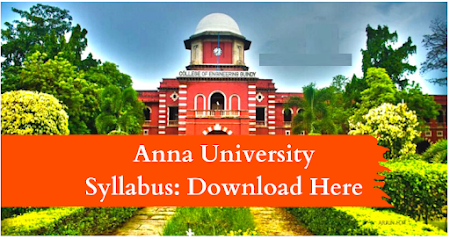### CS8451    DESIGN AND ANALYSIS OF ALGORITHMS     L T P C3 0  0  3

OBJECTIVES:  To understand and apply the algorithm analysis techniques.  To critically analyze the efficiency of alternative algorithmic solutions for the same problem  To understand different algorithm design techniques.  To understand the limitations of Algorithmic power.

UNIT I    INTRODUCTION                                                                                                            9 Notion of an Algorithm – Fundamentals of Algorithmic Problem Solving – Important Problem Types – Fundamentals of the Analysis of Algorithmic Efficiency –Asymptotic Notations and their properties. Analysis Framework – Empirical analysis - Mathematical analysis for Recursive and Non-recursive algorithms - Visualization

UNIT II  BRUTE FORCE AND DIVIDE-AND-CONQUER                                                       9 Brute Force – Computing an – String Matching - Closest-Pair and Convex-Hull Problems - Exhaustive Search - Travelling Salesman Problem - Knapsack Problem - Assignment problem. Divide and Conquer Methodology – Binary Search – Merge sort – Quick sort – Heap Sort - Multiplication of Large Integers – Closest-Pair and Convex - Hull Problems.

UNIT III  DYNAMIC PROGRAMMING AND GREEDY TECHNIQUE                                 9 Dynamic programming – Principle of optimality - Coin changing problem, Computing a Binomial Coefficient – Floyd‘s algorithm – Multi stage graph - Optimal Binary Search Trees – Knapsack Problem and Memory functions. Greedy Technique – Container loading problem - Prim‘s algorithm and Kruskal's Algorithm – 0/1 Knapsack problem, Optimal Merge pattern - Huffman Trees.

UNIT IV  ITERATIVE IMPROVEMENT                                                                                     9 The Simplex Method - The Maximum-Flow Problem – Maximum Matching in Bipartite Graphs, Stable marriage Problem.

UNIT V          COPING WITH THE LIMITATIONS OF ALGORITHM POWER                  9 Lower - Bound Arguments - P, NP NP- Complete and NP Hard Problems. Backtracking – n-Queen problem - Hamiltonian Circuit Problem – Subset Sum Problem. Branch and Bound – LIFO Search and FIFO search - Assignment problem – Knapsack Problem – Travelling Salesman Problem - Approximation Algorithms for NP-Hard Problems – Travelling Salesman problem – Knapsack problem.

TOTAL: 45 PERIODS
OUTCOMES: At the end of the course, the students should be able to:  Design algorithms for various computing problems.  Analyze the time and space complexity of algorithms.  Critically analyze the different algorithm design techniques for a given problem.  Modify existing algorithms to improve efficiency.

TEXT BOOKS:
1. Anany Levitin, ―Introduction to the Design and Analysis of Algorithms‖, Third Edition, Pearson Education, 2012.
2. Ellis Horowitz, Sartaj Sahni and Sanguthevar Rajasekaran, Computer Algorithms/ C++, Second Edition, Universities Press, 2007.

REFERENCES:
1. Thomas H.Cormen, Charles E.Leiserson, Ronald L. Rivest and Clifford Stein, ―Introduction to Algorithms‖, Third Edition, PHI Learning Private Limited, 2012.
2. Alfred V. Aho, John E. Hopcroft and Jeffrey D. Ullman, ―Data Structures and Algorithms‖, Pearson Education, Reprint 2006.
3. Harsh Bhasin, ―Algorithms Design and Analysis‖, Oxford university press, 2016.
4. S. Sridhar, ―Design and Analysis of Algorithms‖, Oxford university press, 2014.
5. http://nptel.ac.in/